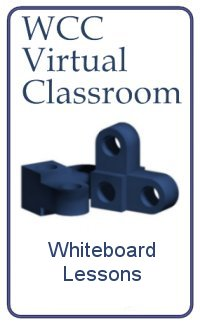Computer-Aided Design Engineering Programming Architectural Design Mechanical Design Civil Design Energy Management Electrical Design

Network
Engineer

Project
Management
 Table of Contents Contemporary Math These lessons in mathematics include recognizing patterns, estimating, exploring sets and portraying them. There are problems that use adding, subtracting, multiplying and dividing fractions and the use of the order of operations. We continue to explore problems involving different numeric systems such as Roman and binary (base 2) numbers. As the quantities increase or decrease greatly, we use scientific notation to handle values to large for the modern calculator. We broaden our horizons with measuring exercises, creating graphs and Venn diagrams. As the topic of contemporary math broadens, we will examine real world applications on this page or point to other web pages specifically on Algebra, Geometry, Trigonometry, and Calculus. Appendix A: Adding Fractions Appendix B: Subtracting Fractions Appendix C: Multiplying Fractions Appendix D: Dividing Fractions Polya Cycle Training Exercise Contemporary Math Quiz 1 Venn Diagrams Contemporary Math Quiz 2 Real World Applications Practice Problems Measuring Lab Measuring and Graphing Lab Real World Applications 1 Contemporary Math Quiz 3 Contemporary Math Final Review